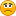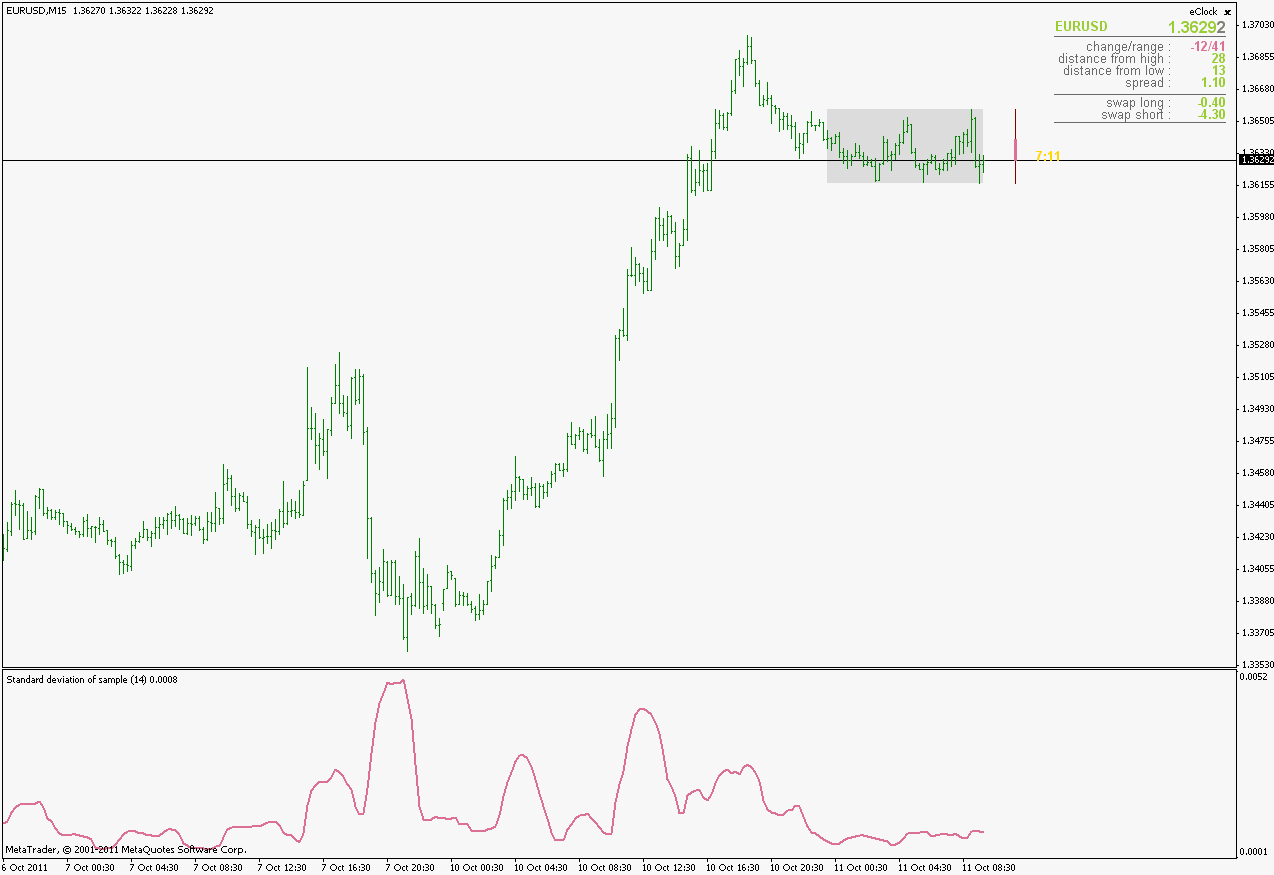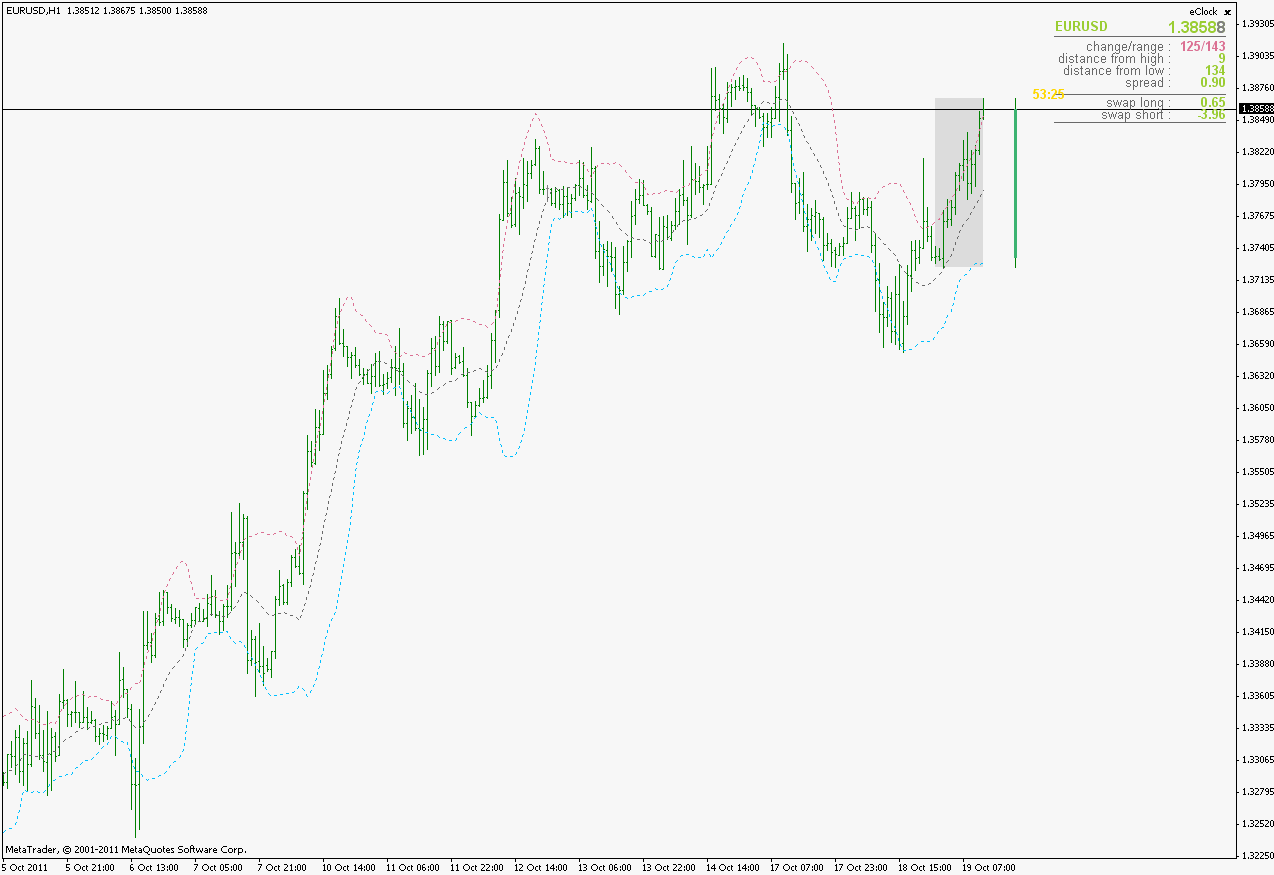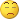Std Deviation - page 41163

Guyver:
I used the word average because with less data there is really no meaningful information we can use through STDEV . So mean or average is fine for the scenario i wrote before imho but in your case

STD DEV..

To get std-dev we need to first get the variance which is another way of saying how the "data" is spread in other words the average of the distance of values from mean squared.. in your example using the same values we can write ( in a basic form ).

// mql4 code

double high = 2.5968;

double low = 2.5706;

double mean = (high+low)/2;

double variance = ((high-low)*(high-low))/2;

double stdev = MathSqrt(variance);

Comment(stdev);

//

As you can see first we take the average or mean cause we need the middle value then we take the variance which is says how the data is spread across mean and then we take the stddev which says how "far"are the typical values from the mean.

-- hope this helps

guyver

Thanks Guyver

That is what I was looking for I should be able to complete the EA now.

Thanks for the help.1152

Beno:
Thanks Guyver

That is what I was looking for I should be able to complete the EA now.

Thanks for the help.

No problem just don't get too much inside the deviation it may take you apart lool. kidding , happy programming!

-guyver1163

Hi attached is the experiment I was working on.

In theory it should work on any pair on the daily TF it uses a version of stdev as the trailing stop and 2 other stdevs formula for the TP and SL thanks to Guyver

The idea was to create a dynamic system based on the what the how far price deviates from the previous bars pivot

Anyway give it a go and post your results or suggestions be them good bad or ugly.

Cheers

Beno

Files:
std.mq4 24 kb4

Was looking for some calculations and when I did not find those for metatrader...

Now, this is made as an indicator but it is rather a tool for calculating certain values for arrays than an indicator

I could not think of a better name for it than deviations (based on the majority of work it does:))

______________________

Parameters are simple except maybe for the modeparameter

Modeparameter values :
0 - A simple moving average (nothing else)

1 - variance (Variance - Wikipedia, the free encyclopedia)

2 - average (absolute) deviation (https://en.wikipedia.org/wiki/Absolute_deviation

3 - standard deviation (Standard deviation - Wikipedia, the free encyclopedia)

4 - skewness (Skewness - Wikipedia, the free encyclopedia)

5 - kurtosis (Kurtosis - Wikipedia, the free encyclopedia)

______________________ As I told, as I could not think of a better name for this and also I did not find a better thread to post it to.

this indicator is the fantastic work。but i HAVE found i can not use the icustom funtion with this indicator. double skewness=iCustom(NULL,TimeFrame,"???(name)",period,price,???(mode),shift);9496

cosmos2011:
this indicator is the fantastic work。but i HAVE found i can not use the icustom funtion with this indicator. double skewness=iCustom(NULL,TimeFrame,"???(name)",period,price,???(mode),shift);

For skewness maybe try iCustom(NULL,0,"Deviations",price,period,4,0,1); or you can in place of 4 put mode then you have choice of calling a different type of the six.4

mrtools:
For skewness maybe try iCustom(NULL,0,"Deviations",price,period,4,0,1); or you can in place of 4 put mode then you have choice of calling a different type of the six.

Thank you very much!151273

Posting this one simply because I like the code of it"Classical" standard deviation calculation algorithm implies that 2 loops are used in its calculation : one when e mean (simple moving average - SMA) is calculated, and the second when the square differences of prices to that mean are calculated. This one does not do it that way, and it does all the necessary calculation in a single loop. Apart from that, it is already ready to calculate standard deviations on any value (no need for a separate "OnArray" version) and it even covers a standard deviation of a sample (of which the built in metatrader standard deviation is completely unaware). So, in the end, this is a kind of an evolution of an indicator, not a revolutionFiles:
std_dev.gif 20 kb505

Simply, I just ad 20,1 to 20,2 on Bollinger Bands and trade between those deviations. The middle part of 20MAwould be a range market. Only look the slope! Horizontal no good.

Cheers Hermes151273

And one more single pass computing way of standard deviation

This one dates way back to 1962 (it was proposed by B. P. Welford) and was described by Donald Knuth in his Art of Computer Programing. This one also has an option to compute standard deviation of a sample (which is by all statisticians the only correct way to calculate standard deviation - no wonder we are having funny results in TA when coders do not listen to the people that invented the math formula for some things)

Attaching also a variation of Bollinger bands indicator that depends largely on standard deviations (even though it is not a thread about Bollinger bands - this version is using by default sample deviation instead of built in deviation) in order to make it possible to compare the results of the built in and this way of standard deviation calculation. Difference is not big, but it is visible even with naked eyeFiles:898

mrtools

mrtools:
Thank you Mladen,here's a picture of AllMa ZeroLag using kurtosis, verry,verry interesting!!!!!

.....and where is that most interesting part ?

I am really missing SOMEthing